MORE IN Engineering Mechanics (EM)
SPPU First Year Engineering (Semester 2)
Engineering Mechanics (EM)
December 2015
Total marks: --
Total time: --
INSTRUCTIONS
(1) Assume appropriate data and state your reasons
(2) Marks are given to the right of every question
(3) Draw neat diagrams wherever necessary

Solve any one question from Q1 and Q2
1 (a) The force system shown in Fig. 1(a) have a resultant of 200 N along positive Y-axis, determine the magnitude and position θ of force F.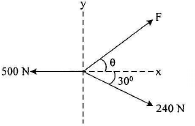4 M
1 (b) Two blocks are connected by an inextensible string as shown in Fig. 1(b). If the system is released from rest, determine the velocity of the block A after it has moved 2 m by work energy principle. The coefficient of friction between block A and the plane is μS=0.25.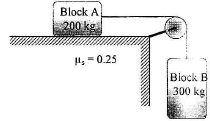4 M
1 (c) A stone is dropped from the top of a tower 50 m high. At the same time, another stone is thrown vertically upwards from the foot of tower with a velocity of 25 m/s. When and where the two stones cross each other?
4 M
1 (d) A cricket ball thrown by a fielder from a height of 2m at an angle of 45° to the horizontal with an initial velocity of 25 m/s hit the wicket at the height of 0.6m from the ground, find distance of fielder from the wickets:
4 M

2 (a) A semicircular area is cut from a trapezium as shown in Fig. 2(a). Determine the centroid of the shaded portion with respect to the origin.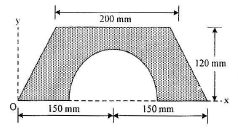4 M
2 (b) A pendulum bob has a mass of 10 kg and is released from rest when θ=0° as shown in Fig. 2(b). Determine the tension in the cord at θ=30°. Neglect the size of bob.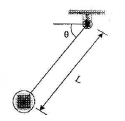4 M
2 (c) A ball is dropped from an unknown height on a horizontal floor from which it rebounds to height of 8 m. If e=0.667, calculate the height from which the ball was dropped.
4 M
2 (d) A bullet moving at a speed of 300 m/s has its speed reduce to 270 m/s when it passes through a board. Determine how many such boards the bullet will penetrate before it stops.
4 M

Solve any one question from Q3 and Q4
3 (a) A simply supported beam AB of span 6m is loaded and supported as shown in Fig. 3(a). Find the reactions at supported A and B.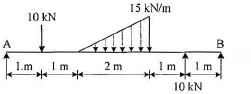6 M
3 (b) Determine the magnitude and direction of a resultant force of a given force system as shown in Fig. 3(b) and locate its point of application on the slab: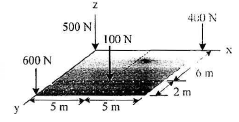6 M
3 (c) A sphere weighing 1000 N is placed in a wrench as shown in Fig. 3(c), find the reactions at the point of contacts: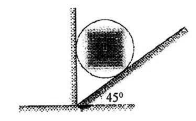5 M

4 (a) Determine the magnitude and position of force F so that the force system shown in Fig. 4(a) maintain equilibrium.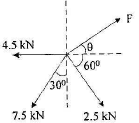6 M
4 (b) If each cable can sustain a maximum tension of 600 N, determine the greatest weight of the bucket and its contents that can be supported. Refer Fig. 4(b).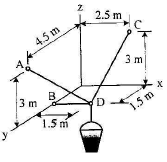6 M
4 (c) Determine the reactions at roller A and pin B for equilibrium of the member ACB as shown in Fig. 4(c).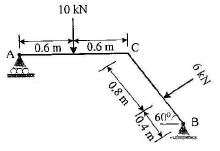5 M

Solve any one question from Q5 and Q6
5 (a) Members AB and BC can support a maximum compressive force of 800 N and members AD, DC and BD can support a maximum tensile force of 2000 N. Determine the greatest load P the truss can support. Refer Fig. 5(a).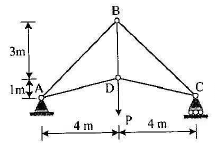6 M
5 (b) The uniform rod having a weight W and length L is supported at its ends A and B as shown in Fig. 5(b), where the coefficient of static friction μs=0.2. Determine the greatest angle θ so that the rod does not slip. Refer Fig. 5(c)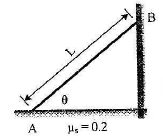6 M
5 (c) Determine the horizontal force P needed to just start moving the 300 N crate up the plane. Take μs=0.3. Refer Fig. 5(c).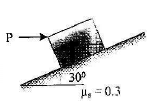5 M

6 (a) Determine the force in the member BE and BD of the truss which supports the load as shown in Fig. 6(a). All interior angles are 60° and 120°.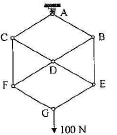6 M
6 (b) Determine the magnitude of pin reactions at A, B and D for the frame loaded as shown in Fig. 6(b).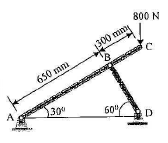6 M
6 (c) A force P=mg/6 is required to lower the cylinder with the cord making 1.25 turns around the fixed shaft. Determine the coefficient of friction μs between the cord and the shaft. Refer Fig. 6(c):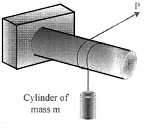5 M

More question papers from Engineering Mechanics (EM)